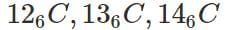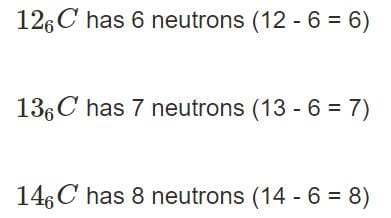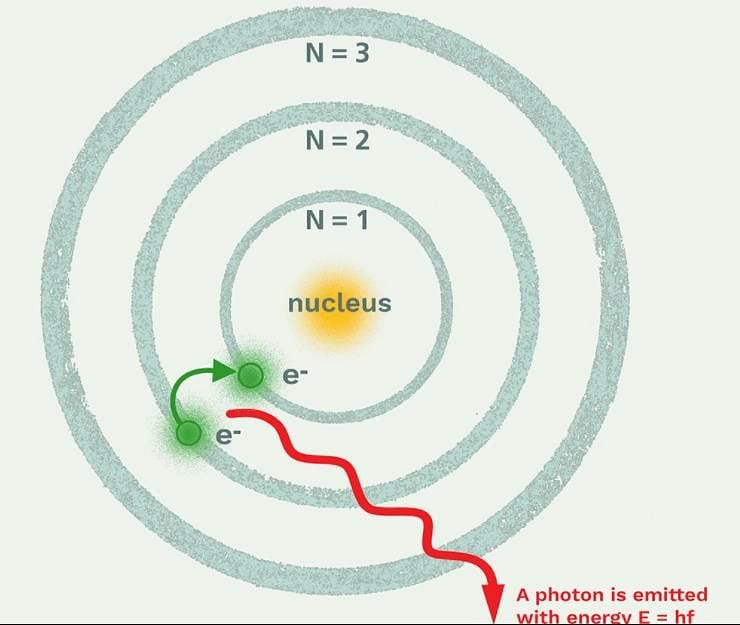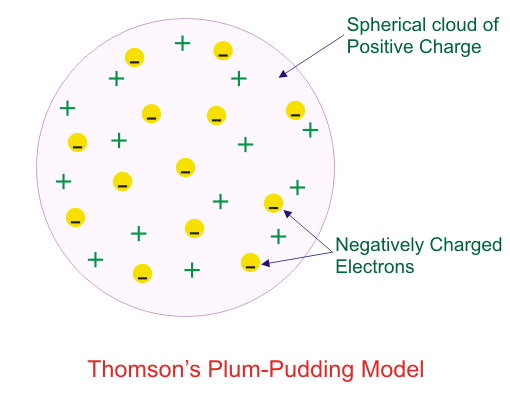NEET  >  Test: Structure of Atom - 1

# Test: Structure of Atom - 1

Test Description

## 15 Questions MCQ Test Chemistry Class 11 | Test: Structure of Atom - 1

Test: Structure of Atom - 1 for NEET 2023 is part of Chemistry Class 11 preparation. The Test: Structure of Atom - 1 questions and answers have been prepared according to the NEET exam syllabus.The Test: Structure of Atom - 1 MCQs are made for NEET 2023 Exam. Find important definitions, questions, notes, meanings, examples, exercises, MCQs and online tests for Test: Structure of Atom - 1 below.
Solutions of Test: Structure of Atom - 1 questions in English are available as part of our Chemistry Class 11 for NEET & Test: Structure of Atom - 1 solutions in Hindi for Chemistry Class 11 course. Download more important topics, notes, lectures and mock test series for NEET Exam by signing up for free. Attempt Test: Structure of Atom - 1 | 15 questions in 15 minutes | Mock test for NEET preparation | Free important questions MCQ to study Chemistry Class 11 for NEET Exam | Download free PDF with solutions
 1 Crore+ students have signed up on EduRev. Have you?
Test: Structure of Atom - 1 - Question 1

### Principle Quantum number describes?

Detailed Solution for Test: Structure of Atom - 1 - Question 1

The principal quantum number (n) describes the size of the orbital.
Example: Orbitals for which n = 2 are larger than those for which n = 1.

In detail Explanation: The principal quantum number is one of four quantum numbers assigned to each electron in an atom to describe that electron's state. Its values are natural numbers (from 1) making it a discrete variable. It is used to describe the size of the orbital.Test: Structure of Atom - 1 - Question 2

### The atoms of different isotopes of the same element differ from each other in the number of:

Detailed Solution for Test: Structure of Atom - 1 - Question 2
• It can be defined as the element which has the same number of protons but differs in neutron number. The atoms of the same element may differ in the number of neutrons but have the same number of electrons. Neutrons are found in the nucleus of the elements and can differ in different isotopes of the same element.

For example:, where 6 which is common among all is atomic number of the element and atomic number is proton number so number of protons is equal and is 6 whereas 12,13 and 14 are mass number which are different and is sum of protons and neutrons. That results in a different neutron number as the proton number is the same.
So, considering the Isotope of C, which has 6 electrons because the atomic number is 6.So, the atoms of various isotopes of a particular element differ from each other in the number of neutrons in the nucleus.
Therefore, the correct answer is option (B).

Test: Structure of Atom - 1 - Question 3

### The radius of the nucleus of an atom is approximately:

Detailed Solution for Test: Structure of Atom - 1 - Question 3

It is found that the radius of the nucleus is approximately 10-15 m.

Test: Structure of Atom - 1 - Question 4

An element has 18 electrons, and 20. neutrons. Its charge is -2. What is its mass number?

Detailed Solution for Test: Structure of Atom - 1 - Question 4
• The mass number of an element is the sum of the number of protons and neutrons in the nucleus of an atom. The number of protons in the nucleus of an atom is equal to the atomic number of the element, which determines the element's identity. The atomic number is also equal to the number of electrons in a neutral atom.

In this case, the element has 18 electrons and a charge of -2, which means it has 2 more electrons than protons. The atomic number of the element is therefore 18 - 2 = 16.

The number of neutrons in the nucleus is 20, and the number of protons is 16. Therefore, the mass number of the element is 20 + 16 = 36.

Test: Structure of Atom - 1 - Question 5

Which of the following statements is not correct about the characteristics of cathode rays?

Detailed Solution for Test: Structure of Atom - 1 - Question 5

The correct answer is Option 4: Characteristics of cathode rays depend upon the nature of gas present in the cathode ray tube. Cathode rays do not depend on the material of electrode and nature of the gas that is filled in the discharged tube. However, the e/m ratio of the charge to mass of the particle is different in the case of anode rays and is dependent on the material of the electrode and the gas present in the discharged tube.

Test: Structure of Atom - 1 - Question 6

Which model describes that there is no change in the energy of electrons as long as they keep revolving in the same energy level and atoms remains stable?

Detailed Solution for Test: Structure of Atom - 1 - Question 6

Bohr Model of atom:

• An atom is made up of three particles: Electrons, neutrons and protons.
• The protons and neutrons are located in a small nucleus at the centre of the atom.
• The electrons revolve rapidly around the nucleus at the centre of the atom.
• There is a limit to the number of electrons that each energy level can hold.
• Each energy level is associated with a fixed amount of energy.
• There is no change in the energy of electrons as long as they keep revolving in the same energy level.

Bohr explained the stability through the concept of revolution of electrons in different energy levels.The change in the energy of an electron occurs when it jumps from lower to higher energy levels. When it gains energy, it excites from lower to higher and vice versa.
Thus energy is not lost and the atom remains stable.

Test: Structure of Atom - 1 - Question 7

The number of radial nodes for 3p orbital is:

Detailed Solution for Test: Structure of Atom - 1 - Question 7
• Number of radial nodes = n - l – 1

where n = principal quantum number, l = azimuthal quantum number

• For 3p orbital, n = 3 – 1 – 1 = 1
• Number of radial nodes = 3 – 1 – 1 = 1.
Test: Structure of Atom - 1 - Question 8

Atomic mass of an element is equal to the sum of?

Detailed Solution for Test: Structure of Atom - 1 - Question 8
• Atomic mass includes the mass of subatomic particles that is protons, neutrons and electrons.
• Generally, atomic mass can be expressed in grams.
• Atomic mass is the sum of the mass of protons, the mass of electrons and mass of neutrons.
• Since the mass of the electron is 1/2000 times lesser than the mass of proton and neutron. Therefore, the electrons do not contribute to the mass of the atom.

Hence the correct answer is C.

Test: Structure of Atom - 1 - Question 9

Thomson’s plum pudding model explained:

Detailed Solution for Test: Structure of Atom - 1 - Question 9
• An atom consists of a positively charged sphere with electrons filled into it. The negative and positive charges present inside an atom are equal and as a whole, an atom is electrically neutral.
• Thomson’s model of the atom as compared to plum pudding and watermelon.
• He compared the red edible part of the watermelon to a positively charged sphere whereas the seeds of watermelon to negatively charged particles.Test: Structure of Atom - 1 - Question 10

Which of the following conclusions could not be derived from Rutherford’s α -particle scattering experiment?

Detailed Solution for Test: Structure of Atom - 1 - Question 10
• Concept of electrons moving in a circular path of fixed energy called orbits was put forward by Bohr and not derived from Rutherford's scattering experiment.
• Out of a large number of circular orbits theoretically possible around the nucleus.
• The electron revolves only in those orbits which have a tired value of energy Hence, these orbits are called energy level or stationary states.
Test: Structure of Atom - 1 - Question 11

The charge on electron was determined by:

Detailed Solution for Test: Structure of Atom - 1 - Question 11

The charge on electrons was determined by Milliken by using an oil drop experiment.

Test: Structure of Atom - 1 - Question 12

The nature of positive rays depends on?

Detailed Solution for Test: Structure of Atom - 1 - Question 12
• The nature of positive rays produced in a vacuum discharge tube depends upon the nature of the gas-filled.
• The positive rays consist of positive ions obtained by removing one or more electrons from gas molecules.
Test: Structure of Atom - 1 - Question 13

The nucleus of a tritium atom, 3H, contains

Detailed Solution for Test: Structure of Atom - 1 - Question 13
• Tritium (3H) is the only radioactive isotope of hydrogen.
• The nucleus of a tritium atom consists of a proton and two neutrons.
Test: Structure of Atom - 1 - Question 14

Which of the following is true about electron?

Detailed Solution for Test: Structure of Atom - 1 - Question 14
• Electrons are negatively charged particles.
• The mass of the electron is 1/2000 times lesser than the mass of proton and neutron. Therefore, the electrons do not contribute to the mass of the atom.
• An electron has an electric charge of -1.602 × 10-19 coulombs) which is equal and opposite to the charge of a proton.
• Electrons are subatomic particles found outside the nucleus, unlike protons and neutrons, which are present inside the nucleus.

Therefore, the correct answer is A.

Test: Structure of Atom - 1 - Question 15

Neutron is a fundamental particle which carries?

Detailed Solution for Test: Structure of Atom - 1 - Question 15
• Proton and electrons are electrically charged, neutrons have no charge they are electrically neutral.
• The mass of a neutron is slightly greater than the mass of a proton,  which is 1 atomic mass unit (AMU).
• (An atomic mass unit equals about 1.67×10−27 kilograms.)

Hence the correct answer is A.

## Chemistry Class 11

199 videos|330 docs|229 tests
Information about Test: Structure of Atom - 1 Page
In this test you can find the Exam questions for Test: Structure of Atom - 1 solved & explained in the simplest way possible. Besides giving Questions and answers for Test: Structure of Atom - 1, EduRev gives you an ample number of Online tests for practice

## Chemistry Class 11

199 videos|330 docs|229 tests

### How to Prepare for NEET

Read our guide to prepare for NEET which is created by Toppers & the best Teachers(Scan QR code)